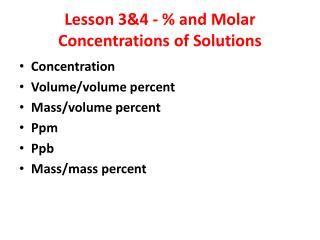Download PresentationLesson 3&4 - % and Molar Concentrations of Solutions

Lesson 3&4 - % and Molar Concentrations of Solutions - PowerPoint PPT Presentation

Lesson 3&4 - % and Molar Concentrations of Solutions. Concentration Volume/volume percent Mass/volume percent Ppm Ppb Mass/mass percent. Concentration. The amount of solute per quantity of solventI am the owner, or an agent authorized to act on behalf of the owner, of the copyrighted work described.
Download PresentationLesson 3&4 - % and Molar Concentrations of Solutions

Download Policy: Content on the Website is provided to you AS IS for your information and personal use and may not be sold / licensed / shared on other websites without getting consent from its author.While downloading, if for some reason you are not able to download a presentation, the publisher may have deleted the file from their server.

- - - - - - - - - - - - - - - - - - - - - - - - - - E N D - - - - - - - - - - - - - - - - - - - - - - - - - -
Presentation Transcript
1. Lesson 3&4 - % and Molar Concentrations of Solutions • Concentration • Volume/volume percent • Mass/volume percent • Ppm • Ppb • Mass/mass percent

2. Concentration • The amount of solute per quantity of solvent • Eg. Beer = 5-9% alcohol, spirits = 40%, Everclear is a colorless and flavorless type of grain alcohol= 95% = illegal in Canada and most States. • Phenol = as little as 1g can be fatal, although it is found in low concentrations in throat spray and lotions to relieve itching.

3. Ppm and Ppb • Ppm – • Ppb – • 1ppm = 1ml of something per 1000L of water • 1ppm = 1mg of something per 1kg of soil • 1ppb = 1ml of something per 1 000 000L of water or 1mg of something per 1000Kg of soil

4. Examples • Lead in drinking water – anything greater than 15ppb is considered hazardous. • Ozone can harm lungs at anything over 0.12ppm

5. Mass/Volume Percent • Unsaturated solutions are often expressed as the mass of solute dissolved per volume of solvent. • Mass/volume percent gives the mass of solute dissolved in a volume of solution, expressed in percent.

6. Sample Problem Mass/Volume • A pharmacist adds 2.0mL of distilled water to 4.00g of a powered drug. The final volume of the solution is 3.00mL. What is the concentration of the drug in g/100mL of solution? What is the percent (m/v) of the solution?

7. Sample Problem (m/v) • Many people use a solution of trisodium phosphate, Na3PO4, (TSP) to clean walls before putting up wall paper. The recommended concentration is 1.7%(m/v). What mass of TSP is needed to make 2.0L of solution?

8. Concentration as a Mass Percent • The concentration of a solution that contains a solid solute dissolved in a liquid solvent can also be expressed as a mass of solute dissolved in a mass of solution. • A mass/mass percent gives the mass of a solute divided by the mass of a solution, expressed as a percent

9. Sample Problem mass/mass % • Calcium chloride, CaCl2, can be used instead of road salt to melt ice on roads. To determine how much calcium chloride was on the road, a student took a sample to analyze. The sample had a mass of 23.47g. When the solution was evaporated, the residue had a mass of 4.58g. What was the mass/mass percent of calcium chloride in the slush? How many grams of CaCl2 were present in 100g of solution?

10. Concentration as a volume/volume % • When mixing two liquids to form a solution, it is easier to measure their volumes than their masses • v/v % gives the volume of solute divided by the volume of the solution, expressed as a percent.

11. Sample Problem v/v% • Rubbing alcohol is commonly used as an antiseptic for small cuts. It is sold as a 70% (v/v) solution of isopropyl alcohol in water. What volume of isopropyl alcohol is used to make 500mL of rubbing alcohol?

12. Molar Concentrations of Solutions • Molar concentration = the number of moles of solute in 1L of solution, also known as molarity. • Molar concentration (mol/L) = amount of solute (mol) / volume of solution (L)

13. Review! Grams to Moles! • Convert 100g of Ca(OH)2 to moles

14. Sample Problem • What is the concentration in moles per litre of a solution containing 0.65mol of NaNO3 in 2500mL of solution? • Given: 0.65 mol NaNO3, 2500mL = 2.5L • Required: mol/L

15. Sample Problem • What is the concentration in moles per litre of a solution containing 49g of sulfuric acid (H2SO4) dissolved in 3.0L of solution? • ANS = 0.17mol/L

16. Sample Problem • A saline solution contains 0.90g of sodium chloride dissolved in 100mL of solution. What is the molar concentration of the solution? • ANS = 0.15mol/L

17. Last Sample Problem • At 20DC a saturated solution of calcium sulphate, CaSO4, has a concentration of 0.0153mol/L. A student takes 65mL of this solution and evaporates it. What mass (g) is left in the evaporating dish?